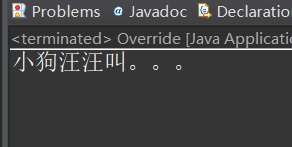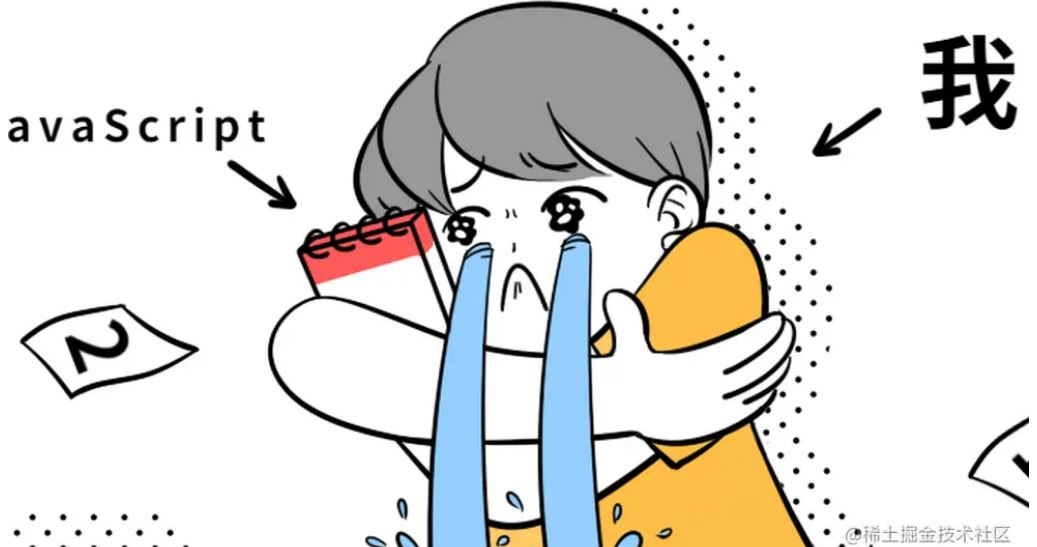# 零基础入门深度学习(5) - 循环神经网络

+关注继续查看

RNN是在自然语言处理领域中最先被用起来的，比如，RNN可以为语言模型来建模。那么，什么是语言模型呢？

基本循环神经网络x是一个向量，它表示输入层的值（这里面没有画出来表示神经元节点的圆圈）；s是一个向量，它表示隐藏层的值（这里隐藏层面画了一个节点，你也可以想象这一层其实是多个节点，节点数与向量s的维度相同）；U是输入层到隐藏层的权重矩阵（读者可以回到第二篇文章《零基础入门深度学习(2) - 神经网络和反向传播算法》，看看我们是怎样用矩阵来表示全连接神经网络的计算的）；o也是一个向量，它表示输出层的值；V是隐藏层到输出层的权重矩阵。那么，现在我们来看看W是什么。循环神经网络的隐藏层的值s不仅仅取决于当前这次的输入x，还取决于上一次隐藏层的值s。权重矩阵 W就是隐藏层上一次的值作为这一次的输入的权重。双向循环神经网络A2和A'2则分别计算：深度循环神经网络循环神经网络的训练算法：BPTT

BPTT算法是针对循环层的训练算法，它的基本原理和BP算法是一样的，也包含同样的三个步骤：

1. 前向计算每个神经元的输出值；

2. 反向计算每个神经元的误差项值，它是误差函数E对神经元j的加权输入的偏导数；

3.  计算每个权重的梯度。------数学公式超高能预警-----------数学公式超高能预警解除-----

RNN的梯度爆炸和消失问题1. 合理的初始化权重值。初始化权重，使每个神经元尽可能不要取极大或极小值，以躲开梯度消失的区域。

2. 使用relu代替sigmoid和tanh作为激活函数。原理请参考上一篇文章《零基础入门深度学习(三) - 卷积神经网络》的激活函数一节。

3. 使用其他结构的RNNs，比如长短时记忆网络（LTSM）和Gated Recurrent Unit（GRU），这是最流行的做法。我们将在以后的文章中介绍这两种网络。

RNN的应用举例——基于RNN的语言模型向量化

1. 建立一个包含所有词的词典，每个词在词典里面有一个唯一的编号。

2. 任意一个词都可以用一个N维的one-hot向量来表示。其中，N是词典中包含的词的个数。假设一个词在词典中的编号是i，v是表示这个词的向量，是向量的第j个元素，则：Softmax层1. 每一项为取值为0-1之间的正数；

2. 所有项的总和是1。

语言模型的训练交叉熵误差RNN的实现

    import numpy as np
from cnn import ReluActivator, IdentityActivator, element_wise_op

    class RecurrentLayer(object):
def __init__(self, input_width, state_width,
activator, learning_rate):
self.input_width = input_width
self.state_width = state_width
self.activator = activator
self.learning_rate = learning_rate
self.times = 0       # 当前时刻初始化为t0
self.state_list = [] # 保存各个时刻的state
self.state_list.append(np.zeros(
(state_width, 1)))           # 初始化s0
self.U = np.random.uniform(-1e-4, 1e-4,
(state_width, input_width))  # 初始化U
self.W = np.random.uniform(-1e-4, 1e-4,
(state_width, state_width))  # 初始化W

        def forward(self, input_array):
'''
根据『式2』进行前向计算
'''
self.times += 1
state = (np.dot(self.U, input_array) +
np.dot(self.W, self.state_list[-1]))
element_wise_op(state, self.activator.forward)
self.state_list.append(state)

def backward(self, sensitivity_array,
activator):
'''
实现BPTT算法
'''
self.calc_delta(sensitivity_array, activator)
def calc_delta(self, sensitivity_array, activator):
self.delta_list = []  # 用来保存各个时刻的误差项
for i in range(self.times):
self.delta_list.append(np.zeros(
(self.state_width, 1)))
self.delta_list.append(sensitivity_array)
# 迭代计算每个时刻的误差项
for k in range(self.times - 1, 0, -1):
self.calc_delta_k(k, activator)
def calc_delta_k(self, k, activator):
'''
根据k+1时刻的delta计算k时刻的delta
'''
state = self.state_list[k+1].copy()
element_wise_op(self.state_list[k+1],
activator.backward)
self.delta_list[k] = np.dot(
np.dot(self.delta_list[k+1].T, self.W),
np.diag(state[:,0])).T
self.gradient_list = [] # 保存各个时刻的权重梯度
for t in range(self.times + 1):
(self.state_width, self.state_width)))
for t in range(self.times, 0, -1):
# 实际的梯度是各个时刻梯度之和
lambda a, b: a + b, self.gradient_list,
'''
计算每个时刻t权重的梯度
'''
self.state_list[t-1].T)
self.gradient_list[t] = gradient

        def update(self):
'''
按照梯度下降，更新权重
'''
self.W -= self.learning_rate * self.gradient

        def reset_state(self):
self.times = 0       # 当前时刻初始化为t0
self.state_list = [] # 保存各个时刻的state
self.state_list.append(np.zeros(
(self.state_width, 1)))      # 初始化s0

    def gradient_check():
'''
梯度检查
'''
# 设计一个误差函数，取所有节点输出项之和
error_function = lambda o: o.sum()
rl = RecurrentLayer(3, 2, IdentityActivator(), 1e-3)
# 计算forward值
x, d = data_set()
rl.forward(x)
rl.forward(x)
# 求取sensitivity map
sensitivity_array = np.ones(rl.state_list[-1].shape,
dtype=np.float64)
# 计算梯度
rl.backward(sensitivity_array, IdentityActivator())
# 检查梯度
epsilon = 10e-4
for i in range(rl.W.shape):
for j in range(rl.W.shape):
rl.W[i,j] += epsilon
rl.reset_state()
rl.forward(x)
rl.forward(x)
err1 = error_function(rl.state_list[-1])
rl.W[i,j] -= 2*epsilon
rl.reset_state()
rl.forward(x)
rl.forward(x)
err2 = error_function(rl.state_list[-1])
expect_grad = (err1 - err2) / (2 * epsilon)
rl.W[i,j] += epsilon
print 'weights(%d,%d): expected - actural %f - %f' % (
i, j, expect_grad, rl.gradient[i,j])Java零基础入门：方法的重写
Java零基础入门：方法的重写
24 0MySQL零基础入门（一）
MySQL零基础入门（一）
23 0🚀从零开始学习JS基础5️⃣🚀

22 0672 05050 02527 01259 03064 07680 0
lhyxcxy

413

0